International
Tables for
Crystallography
Volume C
Mathematical, physical and chemical tables
Edited by E. Prince

International Tables for Crystallography (2006). Vol. C, ch. 2.2, pp. 34-35

Section 2.2.4. Weissenberg geometry

J. R. Helliwella

aDepartment of Chemistry, University of Manchester, Manchester M13 9PL, England

2.2.4. Weissenberg geometry

| top | pdf |

Weissenberg geometry (Weissenberg, 1924) is dealt with in the books by Buerger (1942) and Woolfson (1970), for example.

2.2.4.1. General

| top | pdf |

The conventional Weissenberg method uses a moving film in conjunction with the rotation of the crystal and a layer-line screen. This allows:

 (a) A larger rotation range of the crystal to be used (say 200°), avoiding the problem of overlap of reflections (referred to in Subsection 2.2.3.4on oscillation photography). (b) Indexing of reflections on the photograph to be made by inspection.

The Weissenberg method is not widely used now. In small-molecule crystallography, quantitative data collection is usually performed by means of a diffractometer.

Weissenberg geometry has been revived as a method for macromolecular data collection (Sakabe, 1983, 1991), exploiting monochromatized synchrotron radiation and the image plate as detector. Here the method is used without a layer-line screen where the total rotation angle is limited to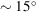; this is a significant increase over the rotation method with a stationary film. The use of this effectively avoids the presence of partial reflections and reduces the total number of exposures required. Provided the Weissenberg camera has a large radius, the X-ray background accumulated over a single spot is actually not serious. This is because the X-ray background decreases approximately according to the inverse square of the distance from the crystal to the detector.

The following Subsections 2.2.4.2and 2.2.4.3describe the standard situation where a layer-line screen is used.

2.2.4.2. Recording of zero layer

| top | pdf |

Normal-beam geometry (i.e. the X-ray beam perpendicular to the rotation axis) is used to record zero-layer photographs. The film is held in a cylindrical cassette coaxial with the rotation axis. The centre of the gap in a screen is set to coincide with the zero-layer plane. The coordinate of a spot on the film measured parallel (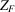) and perpendicular (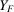) to the rotation axis is given by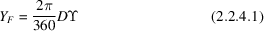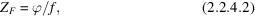whereis the rotation angle of the crystal from its initial setting, f is the coupling constant, which is the ratio of the crystal rotation angle divided by the film cassette translation distance, in ° min−1, and D is the camera radius. Generally, the values of f and D are 2° min−1 and 28.65 mm, respectively.

2.2.4.3. Recording of upper layers

| top | pdf |

Upper-layer photographs are usually recorded in equi-incli­nation geometry [i.e. μ = −ν in equations (2.2.3.7)and (2.2.3.8)]. The X-ray-beam direction is made coincident with the generator of the cone of the diffracted beam for the layer concerned, so that the incident and diffracted beams make equal angles (μ) with the equatorial plane, where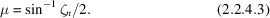The screen has to be moved by an amount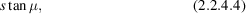where s is the screen radius. If the cassette is held in the same position as the zero-layer photograph, then reflections produced by the same orientation of the crystal will be displaced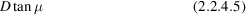relative to the zero-layer photograph. This effect can be eliminated by initial translation of the cassette by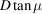.

References

Buerger, M. J. (1942). X-ray crystallography. New York: John Wiley.
Sakabe, N. (1983). A focusing Weissenberg camera with multilayer-line screens for macromolecular crystallography. J. Appl. Cryst. 16, 542–547.
Sakabe, N. (1991). X-ray diffraction data collection systems for modern protein crystallography with a Weissenberg camera and an imaging plate using synchrotron radiation. Nucl. Instrum. Methods, A303, 448–463.
Weissenberg, K. (1924). Ein neues Röntgengoniometer. Z. Phys. 23, 229–238.
Woolfson, M. M. (1970). Introduction to X-ray crystallography. Cambridge University Press.